﻿
Reach Us+44-7482-875032
Energy is Not Conserved

# Journal of Applied & Computational MathematicsOpen Access

All submissions of the EM system will be redirected to Online Manuscript Submission System. Authors are requested to submit articles directly to Online Manuscript Submission System of respective journal.

# Energy is Not Conserved

GuagSan Yu*
Department of Mathematics, Harbin Normal University, Dynamics Institute, Shida Road, Limin Economic Development Zone, Harbin City, P.R. China
*Corresponding Author: GuagSan Yu, Department of Mathematics, Harbin Normal University, Dynamics Institute, Shida Road, Limin Economic Development Zone, Harbin City, P.R. China, Tel: +86 451 8806 0555, Email: [email protected]

Received Date: May 09, 2018 / Accepted Date: May 29, 2018 / Published Date: Jun 10, 2018

Keywords: Energy; Force; Newton's law; New law of motion; Energy is not conserved PACS: 45.20.Dd, 45.40.àf, 45.50.àj, 45.50.Dd

#### Introduction

Newton's third law proved to be wrong , the new third law of motion shows, that the object and the object its force and reaction force, in most cases is different. From the force and reaction force is not the same, Can prove that energy is not conserved.

#### In Newton's Third law Energy Is Not Conserved!

In Newton's third law, the energy is not conserved, which must be shocking, but it is actually the case .

According to Newton's third law, the force and the reaction force is equal magnitude and opposite direction . Then assuming that there are two different mass the objects, they to be interaction, so the force should be:

-F1⇔ F2 (1)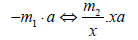(2)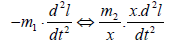(3)

Force and reaction force of the role of time is the same , so the above two objects interact with each mobile distance should be, if m1 is the distance l then m2 is xl.

So the force and reaction force, by does work is as: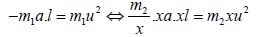(4)

In the above analysis , we assume that the mass of the two objects is different. For example, the mass of object 2 is 1/x of mass of object 1. Therefore, in the above formula, m1 and m2 are actually of the same mass. So the two objects after interaction , the resulting kinetic energy is different:

-m1 u2<m2 xu2 (5)

In fact the kinetic energy of the two objects in opposite directions, so the total kinetic energy at this 

time should be:

m1 u2+m2 xu2=m( x-1) u2 (6)

If the interaction of the above two objects, is formed by the movement of the object 1 and the stationary object 2 in impact .

Then, after the impact, the total kinetic energy of the two objects occurs as shown in the formula (6) the value change.

Assuming that the interaction of the two objects is fully elastic, then the two objects have only the mechanical energy and kinetic energy of the influence, represented by formula (6), is the role of the above process all the energy .

So now let us look at the above process only two objects of mutual impact, that is, the two objects are not subject to the role of other external forces, not subject to objects outside other the energy impact. Therefore, according to the law of conservation of energy, the two the total energy state of the object should remain the same .

However, according to the formula (6), the two objects after the above process, it has indeed occurred in its total kinetic energy changes.

-m1 u12+m2 xu2 ⇒ m (x-1) u2 (7)

So the two mass different of objects in after the impact, the total kinetic energy has changed, so they are the energy is not conservative.

So even the Newton's law and the classical mechanics of the functional principles can also be found in the energy is not conservation of the situation.

#### New Law of Motion the Energy is not Conserved

In the case of the new second and new third law of motion , because the dynamic force and the dynamic reaction force are usually different, so the two objects after interact, the total kinetic energy will be greater change, so that the energy is not conserved the situation will be more salience and more intense.

#### New Second and New Third Movement Law and Kinetic energy

In the new law of motion, the concept of kinetic energy should be different. In the tradition theory, the kinetic energy is also called the work . Is the product of the force and distance.

W = F ⋅l (8)

But in fact it is incredible. Because of the movement of matter, the force F itself has the property of the movement of matter and matter and the movement change, and when it is multiplied by a distance l, the unit of movement will be power. So what can it be that, do it is a correct reflection of the energy of the material movement?

On the new law of motion, on which the concept of kinetic energy, may wish to learn about the expression of electricity, because the two have similarities, such as the concept of electrical power is :

W =V ⋅ I I (9)

That is, the electric power W is equal to the voltage V multiplied by the current I. And in mechanics, the force F is equal to the mass m of the object multiplied by the acceleration a. So both of which are very similar.

F = m⋅ a (10)

Where the electric power W of the electric energy intensity is correspond to the force F indicating the strength of the mechanical energy. And the mass m in the mechanical energy is correspond to the voltage V in the electric power, and the acceleration a is correspond to the current I. In the electric power, of the consumption of electricity is the electric energy, it is equal to the electric power multiplied by the time elapsed.

W ⋅t =V ⋅ I ⋅t t (11)

Then in the mechanics of mechanical energy measurement, but also to force be multiplied by time, cannot it?

F ⋅t = m⋅ a ⋅t (12)

Obviously this is the impulse of force in mechanics.

Can the impulse of the force be used to characterize the energy of the kinetic energy of the movement of the object? The answer is that it should be, and still be the most suitable.

Because according to the expression of the new second law of motion [1,2]: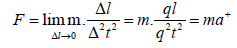(13)

And the new second law of motion of the identical transform [1,2] :

F=βm.β-2 a+ (14)

When using a spring, two times to release respectively impel the two different mass of the object. To the two objects formed the impulse and momentum is the same. Which is: ql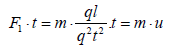(15)

And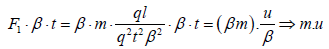(16)

The two times to releases of a spring, are clearly considered to be two equally force and two the same kinetic energy. And then it acts on different mass objects, to form the same the impulse and momentum, what is it? Obviously, it shows that the impulse and momentum formed by the action of the object are proportional to the force and kinetic energy of the object.

So that the momentum of the object is representative of the kinetic energy of the object, it should not be wrong.

So the correct expression for the kinetic energy of an object should be:

W=F. t=m.u (17)

Therefore the kinetic energy of an object equals the momentum of an object.

It may be said that the work of the force on the object, the force and the distance of the product is suitable for the expression, when the force is used to overcome the friction, and the object when the move. Then in fact this is not the case. Because the object of the movement, must be closely linked with the speed of time, so even if the object to overcome the friction and move the situation, but also the force and force used time to calculate be characterized.

The New second and new third law with energy is not conserved

As mentioned earlier, in the case of Newton's law of motion, the role and the reaction there is also have of kinetic energy is not conserved.

In the case of the new second and the new third law of motion, the energy and kinetic energy are not conserved and will be more intense.

For example, when two different mass objects interact: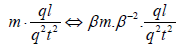(18)

Because the role of time should be the same, so the formula a side to do identity transform: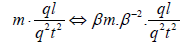(19)

So the two sides of the object the impulse and momentum is: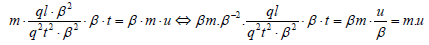(20)

The momentum on the left than the right Big a multiple of β. The previous section mentions that the momentum of the movement of the object is actually the kinetic energy of the object. So the above two objects interact, by action force to two objects export the kinetic energy, the both of difference a multiple of β.

If the interaction of two different objects is formed in the space by mutual collision. Then the force on the two objects, in the opposite direction of the output of the kinetic energy is not the same. So after the action, the total momentum and kinetic energy of two the object, in the space necessity change. And the total kinetic energy of the two objects before and after the collision is changed, it is not the same.

Therefore, the energy is not conserved, will occur in two different mass objects, interaction in space. When two objects are completely elastic collide with each other, only mechanical energy transfer and conversion between the two objects. Therefore, the energy in this case is not conserved, there is absolutely no doubt about it.

Therefore, as shown below, when the collision between two objects, the kinetic energy changes occur when different, then:

W1W2 (21)

And ( W1=F1.tx = ͎β.m.u) > (W2 =F2.tx=m. u ) (22)

Therefore W1 ±W2≠ 0 (23)

This means that after the collision of two objects, their total kinetic energy has changed.

Because in the above process, no external force on the two objects, while the total kinetic energy of the two objects have changed, so here the energy is not conserved.

#### Energy and Kinetic Energy is not Conserved the Specialty

The aforementioned energy is not conservation namely kinetic energy is not conservation, obviously this is mainly due to, the role of the two objects of different mass, so the interaction between the two is also different. Thus further lead to different impulse, different momentum, and different kinetic energy.

F=βm.β-2a+ (24)

F1.βt=m.a+.βt=β.m.u⇔F2.βt=βm.β-2a+.βt=m.u (25)

Namely F1.βt=β.m.u⇔F2.βt ⇔F2.βt=m.u (26)

So the two objects in the mass is big that, by the small force. Small mass the objects, by the big force. Big mass the objects and small mass the objects, get the size of the dynamic force by the ratio, and big mass the object and small mass the object between the mass ratio is inverse ratio.

So if m1= m m2=βm (27)

Then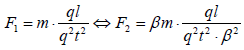(28)

Therefore, the two objects interact, the mass ratio, is its force, impulse, momentum namely kinetic energy inversely proportional.

So the two objects interact, when the mass of the two objects is different and the difference is large, the mass of small objects will be get a great force and the role of impulse, forming a lot of momentum and kinetic energy. And that the mass big of objects, it is subject to a small force and impulse effect, the formation of momentum and kinetic energy is very small.

So when two different mass the objects, in after the action, they in the opposite direction, the formation of momentum and kinetic energy and its changes, that represents the two objects, the total kinetic energy in after its interaction, the changes that have happen. So the energy (kinetic energy) is not conserved.

So in this energy is not conservation, it is possible that the energy becomes larger mass larger objects with small mass objects to collision; it is also possible that energy becomes smaller a small mass of objects with large mass of objects to collision. So the energy it is may become larger, also may become smaller. Energy is not conservative.

Because in accordance with the new second and new third law of motion, the momentum of the object it is its kinetic energy. So the kinetic energy is not conserved, namely that momentum is not conserved. And on the momentum is not conserved, in the previous paper on the new law of motion, also to prove in advance.

Energy is not conserved of the situation, in the traditional power machinery, is also possible existence. Such as electromagnetic generators, the mechanism of its operation is, the conductor cuts the magnetic field lines to generate current. And in this, whatever is the magnetic field, or the rotation of the armature, compared with the current generated in the armature, are equivalent to have great mass. And the current, the smallest unit is the electron in the conductor, it can therefore be considered that, is a very small mass. Therefore, the usual electromagnetic generators, its operation of the power generation process, may be a process of increasing energy. That is, the power generated by the generator, greater than the kinetic energy consumed by its operation.

Opposite of this, the usual electric motors, is the current in the conductor, forming a magnetic field around its conductor, and then use the magnetic field to promote the motor rotation. Then the current in the conductor, is a collection of dense electrons, it and with the magnetic field generated by it, have with a macroscopic form, so it is not necessary to be considered to have a small mass. So the operation of electric motors, is not necessarily a process of diminish energy. Its energy consumption, may have a relatively high performance.

So use good performance the generator, send electricity to promote a good performance the motor. And this motor, it is also used to push the generator to run. So the combination of these two generators and motors, it may constitute a perpetual motion machine. Miracle that is produced.

Energy and kinetic energy is not conservation, may also affect the operation of celestial bodies in the universe. Such as a spherically distributed, dense, rotating cluster, when there is a small amount of interstellar material close to the part of the rotation axis of the cluster, moving away from the outer circumference orientation of the cluster away from the cluster rotation axis, it is compared with the mass of all other substances in the cluster, is very small. So it is in the cluster of all the other great material under the action, when it is closer to the outer circumference of the cluster, it rotates around the cluster to the faster. In the process, this small of cluster material, from the surrounding other giant clusters of material, by the role of force, and increase its speed. On the contrary the individual of the cluster material, relative to other clusters of material collection, mass is relatively very small. So it to the reaction of other clusters material, it is very small and can be ignored.

Can be calculated at this time the total angular velocity of the cluster changes :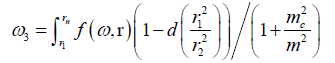(29)

Because the composite mass mc of the total matter of the cluster is extremely large, and the mass m of moving material in the cluster is relatively very small, so when the cluster of the rotation of the total angular velocity changes ω 3, it will be extremely small so it is negligible.

When countless matter individual from this globular cluster, respectively from the direction of the rotation axes of the cluster, and moves to the outer circumferential direction away from the rotation axis direction. It can be from rotated of a spherical star cluster, eventually becoming a thin discshaped rotating cluster. Because in all these processes, the overall star cluster to get reaction, is always relatively small can be ignored. So to complete the above changes in the form of star clusters, the total angular velocity of the cluster is almost constant, and it has more clusters of material, moved to the direction of the cluster rotation radius r be more, so the total kinetic energy of the star cluster, but continue to become more and more [4-7].

So the interstellar material in the universe, its total movement of energy, is also able to change. it may become bigger and bigger, it also may become smaller and smaller.

Because the energy can change, mass big objects act on objects of small mass, energy can become larger; on the contrary, energy can become smaller. Therefore, in the use of power machinery strategy, do some deliberate choice, may be useful. For example, with a powerful power of the engine, driven to light mechanical operation, its energy use efficiency may be greater. If it is weak with the power, driven to more heavy mechanical operation, its energy use efficiency may be very low [8-10].

Therefore, according to the principle of energy is not conserved, human in use energy, it is possible to make energy more and more. Even in theoretically, in the past has been the world's laughing perpetual motion machine, it is not impossible [11-13]. When the miracle will happen.

#### Summingup

Energy is not conserved. According to the principle of energy is not conserved, countless scientific miracles are possible to achieve.

#### Acknowledgements

The authors to thank the Editorial Office. The authors to thank references author. The authors to thank his teachers, is for his technological campaign by gave the sustain: Professor Shixu Guan, Chief editor Xinmin Zhu, Principal Lanxu Xu. The authors thank do ever assisted his the university: Department head Shuquan Wang, Department head Xinde Jiang, Associate Professor Risheng Piao. And many teachers.

#### References

Citation: Yu G (2018) Energy is Not Conserved. J Appl Computat Math 7: 406. DOI: 10.4172/2168-9679.1000406

Copyright: © 2018 Yu G. This is an open-access article distributed under the terms of the Creative Commons Attribution License, which permits unrestricted use, distribution, and reproduction in any medium, provided the original author and source are credited.

Select your language of interest to view the total content in your interested language

##### Recommended Journals
Viewmore
###### Article Usage
• Total views: 666
• [From(publication date): 0-2018 - Aug 24, 2019]
• Breakdown by view type
• HTML page views: 620
• PDF downloads: 46

## Post your commentCan't read the image? click here to refresh
###### Peer Reviewed Journals

Make the best use of Scientific Research and information from our 700 + peer reviewed, Open Access Journals

International Conferences 2019-20

Meet Inspiring Speakers and Experts at our 3000+ Global Annual Meetings

Top